# Touching Up Another Old Photo With OpenCV

So, this (image file of a) photo is, allegedly, of my grandfather's grandfather, Abraham Snyder. Who alleges this, you ask? Well, I don't really remember, but let's leave questions of provenance for another time. The point is, while it's neat to have, it's not in tip-top shape, and it's another opportunity for me to play with the OpenCV library.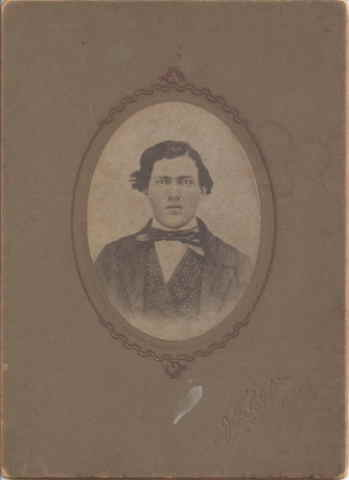So, the first thing was to convert it to black and white, since there really isn't any color information in it anyway (you can always return the final product to sepia-tone if you want). Then, we want to crop it to the oval-shaped part of the image that we really care about (so that later processing isn't impacted by what's outside the oval). This is pretty easy to do with OpenCV, and the result looks like this: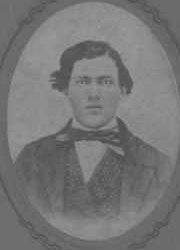``` import cv2 from matplotlib import pyplot as plt import numpy as np img_path = "AbrahamSnyder.jpeg" img = cv2.imread(img_path, cv2.IMREAD_GRAYSCALE) #approximately speaking, this box contains the oval of the actual picture img = img[100:350,80:260] images = [(img,"BW and Cropped")] ```

Better than just cropping it to a rectangle, though, is to actually mask out everything outside the oval-shaped region of the actual picture.``` mask = np.zeros(img.shape[:2], dtype="uint8") #approx center of oval is now 90,120, as are the lengths of the oval's axes cv2.ellipse(mask, (90, 120), (90, 120), 0, 0, 360, 128, -1) masked = cv2.bitwise_and(img, img, mask=mask) images.append((masked,"Masked")) ```

It looks pretty noisy, so we can use OpenCV's "de-noise" function to try to address that. You have to mess around with it a while to find out how much it should be doing; I settled on a value that I thought was good for removing most of the noise, but preserving facial features well. As we'll see in a bit, I came to have second thoughts about this later.``` denoi = cv2.fastNlMeansDenoising(masked, None, 4) <-- value of 4 is a parameter to say how much to "smear" to get rid of noise images.append((denoi,"Denoised")) ```

So now, we can try to improve the contrast. The first thing I tried was "Histogram Equalization" (details), which was what worked well for another old picture of my ancestors as detailed in my last post. Here, though the results were not quite so desirable.``` equ = cv2.equalizeHist(denoi) images.append((equ,"Histogram Equalized")) ```

It seems to have gone a bit overboard on Abraham's forehead, in particular. So, there is a variant of this called "Contrast Limited Histogram Equalization", which seems to address this. It's more complex in theory, but not actually all that much harder to program using OpenCV.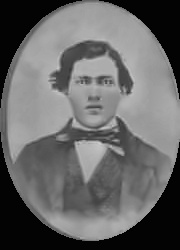``` clahe = cv2.createCLAHE(clipLimit=2.0, tileGridSize=(8,8)) cl1 = clahe.apply(denoi) images.append((cl1,"Contrast Limited Hist Eq")) ```

There's also an alternative method, called Gamma Transform, which does about as well, although you have to do a lot of experimenting to find the right value of gamma.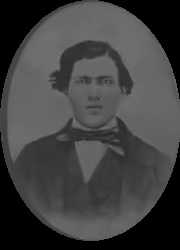``` gamma = 1.5 <-- this value found purely empirically, but trying a bunch of values and seeing what looked the best gam_t = np.array(255*(denoi / 255) ** gamma, dtype = 'uint8') images.append((gam_t,"Gamma Transformed")) ```

At about this time, I took a closer look at Abraham's waistcoat, as shown in the original image, before I "de-noised" it.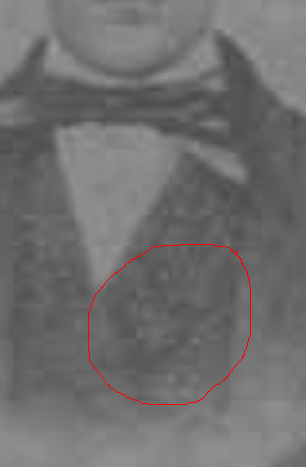Hmmm...that looks like some kind of brocade pattern, which the denoising has completely removed. That's the issue with denoising; it doesn't necessarily know how to distinguish "noise" from "complex pattern". So, I guess what we would like to do is run the denoising on everything except the waistcoat? I played around with the OpenCV "grabcut" method, but it had a hard time distinguishing the waistcoat from the rest of Abraham, and I still wanted the denoising on his face and the light background. So, I decided instead to take advantage of the fact that in python, images are just arrays of numbers, in other words they're just integers that you can do math on. Basically, anywhere it was light colored (we use a cutoff value of 120 here), we wanted to use the denoised image. Everywhere else, we wanted to keep the original (so that we could keep the waistcoat's brocade pattern, if that's what it is).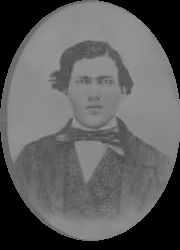Using this as the base, we can again apply the CLAHE transform to get our best result.Here's the full code, from start to finish, for all of the affects.

``` import cv2 from matplotlib import pyplot as plt import numpy as np PARTIAL_DENOISE = True <-- set to False if you don't want to exclude dark areas from the denoising img_path = "AbrahamSnyder.jpeg" img = cv2.imread(img_path, cv2.IMREAD_GRAYSCALE) #approximately speaking, this box contains the oval of the actual picture img = img[100:350,80:260] images = [(img,"BW and Cropped")] mask = np.zeros(img.shape[:2], dtype="uint8") #approx center of oval is now 90,120, as are the lengths of the oval's axes cv2.ellipse(mask, (90, 120), (90, 120), 0, 0, 360, 128, -1) masked = cv2.bitwise_and(img, img, mask=mask) images.append((masked,"Masked")) denoi = cv2.fastNlMeansDenoising(masked, None, 4) if PARTIAL_DENOISE: #use "denoi" image where it is light colored, elsewhere use "masked" image denoi = np.where((denoi>120),denoi,masked).astype('uint8') images.append((denoi,"Part Denoised")) else: images.append((denoi,"Denoised")) equ = cv2.equalizeHist(denoi) images.append((equ,"Histogram Equalized")) clahe = cv2.createCLAHE(clipLimit=2.0, tileGridSize=(8,8)) cl1 = clahe.apply(denoi) images.append((cl1,"Contrast Limited Hist Eq")) gamma = 1.5 gam_t = np.array(255*(denoi / 255) ** gamma, dtype = 'uint8') images.append((gam_t,"Gamma Transformed")) for i,im in enumerate(images): plt.subplot(2,3,i+1).set_title(im) plt.imshow(im, cmap="gray", interpolation="nearest") file_name = "AbrahamSnyder"+im.replace(" ","") if PARTIAL_DENOISE: file_name += "_v2.jpg" else: file_name += ".jpg" cv2.imwrite(file_name,im) plt.show() ```

There are, of course, additional possibilities here. We could make a mask that includes ONLY the waistcoat, so that the suitcoat would still get denoised. On the other hand, is that noise, or a striped pattern on the coat? This is one of the reasons that it is difficult to fully automate photo touchup like this; you need to know something about what you're looking at to tell which transform or effect is the one that is most accurate. I never met my grandfather's grandfather, so I cannot say what his taste in suitcoats was. But, I would definitely wear that waistcoat.